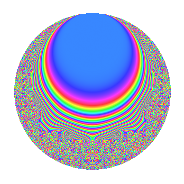# Properties

 Label 15.10.eLevel 15 Weight 10 Character orbit e Rep. character $$\chi_{15}(2,\cdot)$$ Character field $$\Q(\zeta_{4})$$ Dimension 32 Newform subspaces 1 Sturm bound 20 Trace bound 0

# Related objects

## Defining parameters

 Level: $$N$$ $$=$$ $$15 = 3 \cdot 5$$ Weight: $$k$$ $$=$$ $$10$$ Character orbit: $$[\chi]$$ $$=$$ 15.e (of order $$4$$ and degree $$2$$) Character conductor: $$\operatorname{cond}(\chi)$$ $$=$$ $$15$$ Character field: $$\Q(i)$$ Newform subspaces: $$1$$ Sturm bound: $$20$$ Trace bound: $$0$$

## Dimensions

The following table gives the dimensions of various subspaces of $$M_{10}(15, [\chi])$$.

Total New Old
Modular forms 40 40 0
Cusp forms 32 32 0
Eisenstein series 8 8 0

## Trace form

 $$32q - 150q^{3} - 4548q^{6} - 9760q^{7} + O(q^{10})$$ $$32q - 150q^{3} - 4548q^{6} - 9760q^{7} - 39820q^{10} + 191940q^{12} + 114260q^{13} - 40230q^{15} - 1494724q^{16} + 994800q^{18} + 592212q^{21} + 308260q^{22} + 5808020q^{25} + 4786830q^{27} - 12953300q^{28} - 17343060q^{30} + 16124104q^{31} + 19638120q^{33} - 48943956q^{36} - 35578660q^{37} + 144132840q^{40} + 46648260q^{42} - 118721020q^{43} - 129688380q^{45} + 3145024q^{46} + 275395140q^{48} - 64226868q^{51} - 157103680q^{52} + 144539740q^{55} + 48163500q^{57} - 209634300q^{58} - 140815620q^{60} + 63607744q^{61} + 89858580q^{63} + 358931400q^{66} + 153311540q^{67} + 420519420q^{70} - 1534046760q^{72} + 628070360q^{73} + 138090210q^{75} - 2457023472q^{76} - 403337880q^{78} + 2250542952q^{81} + 4138031440q^{82} + 108907340q^{85} - 2878139760q^{87} - 595851300q^{88} + 693868920q^{90} - 4369040216q^{91} - 293672640q^{93} + 7291125636q^{96} + 3987642200q^{97} + O(q^{100})$$

## Decomposition of $$S_{10}^{\mathrm{new}}(15, [\chi])$$ into newform subspaces

Label Dim. $$A$$ Field CM Traces $q$-expansion
$$a_2$$ $$a_3$$ $$a_5$$ $$a_7$$
15.10.e.a $$32$$ $$7.726$$ None $$0$$ $$-150$$ $$0$$ $$-9760$$

## Hecke characteristic polynomials

There are no characteristic polynomials of Hecke operators in the database